# Question Bank Solutions for BE Mechanical Engineering Semester 1 (FE First Year) - University of Mumbai - Engineering Mechanics

Subjects
Topics
Subjects
Popular subjects
Topics
Engineering Mechanics
< prev  21 to 40 of 84  next >

Two blocks A and B are resting against the wall and floor as shown in the figure.Find the minimum value of P that will hold the system in equilibrium. Take μ=0.25 at the floor,μ=0.3 at the wall and μ=0.2 between the blocks.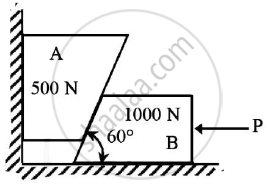Given : μ=0.25 at floor
μ=0.2 between blocks
To find : Minimum value of force P

 Equilibrium of System of Coplanar Forces
Chapter:  Equilibrium of System of Coplanar Forces
Concept: Condition of equilibrium for concurrent forces

For the truss shown in figure 4, find: (i) zero force members, if any (Justify your answer with FBD), (ii) support reactions at C and D.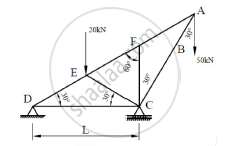Types of Support
Chapter:  Types of Support

For the composite lamina shown in the figure, determine the coordinates of its centroid.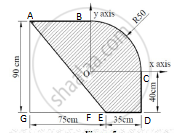Center of Gravity and Centroid for Plane Laminas
Chapter:  Center of Gravity and Centroid for Plane Laminas
Concept: Centroid for Plane Laminas
Replace the force system shown in Figure 6 with a single force and couple system acting at point B.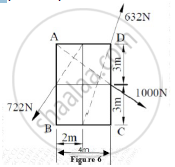[6.01] Resultant of Noncoplanar force systems
Chapter: [6.01] Resultant of Noncoplanar force systems
Concept: Resultant of Concurrent Force System

Using Principle of Virtual Work, determine the force P which will keep the weightless bar AB in equilibrium. Take length AB as 2m and length AC as 8m. The bar makes an angle of 30° with horizontal. All the surfaces in contact are smooth. Refer Figure 9.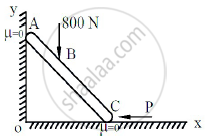Principle of Virtual Work
Chapter:  Principle of Virtual Work
Concept: Applications on Equilibrium Mechanisms

Three forces F1, F2 and F3 act at the origin of Cartesian coordinate axes system. The force F1 (= 70N) acts along OA whereas F2 (= 80N) acts along OB and F3 (= 100N) acts along OC. The coordinates of the points A, B and C are (2,1,3), (-1,2,0) and (4,-1,5) respectively. Find the resultant of this force system.

[6.01] Resultant of Noncoplanar force systems
Chapter: [6.01] Resultant of Noncoplanar force systems
Concept: Resultant of Concurrent Force System

For the truss shown in Figure 16, find the forces in members DE, BD and CB.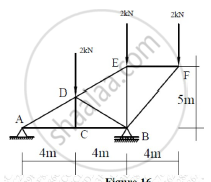Types of Support
Chapter:  Types of Support

Find the co-ordinate of the centroid of the area as shown in the given figure.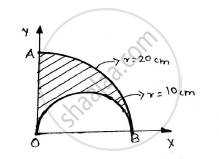Kinematics of Particle
Chapter:  Kinematics of Particle
Concept: Velocity and Acceleration in Terms of Rectangular Co-ordinate System

A force of 500 N is acting on a block of 50 kg mass resting on a horizontal surface as shown in the figure. Determine the velocity after the block has travelled a distance of 10m. Co efficient of kinetic friction is 0.5.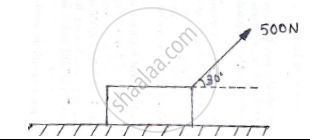Friction
Chapter:  Friction
Concept: Cone of Friction

The position vector of a particle which moves in the X-Y plane is given by 𝒓̅ = (3t3-4t2)𝒊̅ + (0.5t4)𝒋̅

 Kinematics of Particle
Chapter:  Kinematics of Particle
Concept: Velocity and Acceleration in Terms of Rectangular Co-ordinate System

Find the resultant of the force acting on the bell crank lever shown. Also locate its position with respect to hinge B.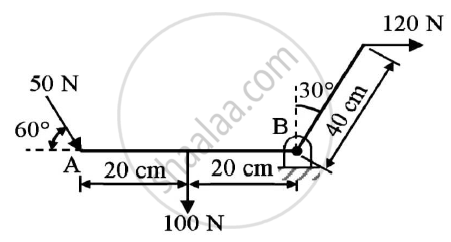Given : Forces on the bell crank lever
To find : Resultant and it’s position w.r.t hinge B

 System of Coplanar Forces
Chapter:  System of Coplanar Forces
Concept: Resultant of parallel forces

Two balls having 20kg and 30 kg masses are moving towards each other with velocities of 10 m/s and 5 m/s respectively as shown in the figure.
If after the impact ,the ball having 30 kg mass is moving with 6 m/s velocity to the right then determine the coefficient of restitution between the two balls.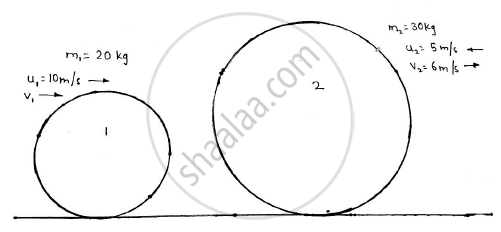Kinematics of Particle
Chapter:  Kinematics of Particle
Concept: Velocity and Acceleration in Terms of Rectangular Co-ordinate System

Find the support reactions at A and B for the beam loaded as shown in the given figure.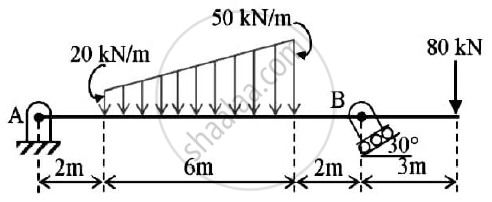Equilibrium of System of Coplanar Forces
Chapter:  Equilibrium of System of Coplanar Forces
Concept: Condition of equilibrium for parallel forces

The V-X graph of a rectilinear moving particle is shown. Find the acceleration of the particle at 20m,80 m and 200 m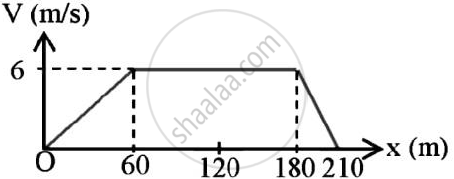Kinematics of Particle
Chapter:  Kinematics of Particle
Concept: Velocity and Acceleration in Terms of Rectangular Co-ordinate System

A bar 2 m long slides down the plane as shown.The end A slides on the horizontal floor with a velocity of 3 m/s.Determine the angular velocity of rod AB and the velocity of end B for the position shown.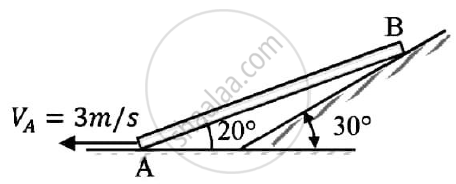Kinematics of Particle
Chapter:  Kinematics of Particle
Concept: Velocity and Acceleration in Terms of Rectangular Co-ordinate System

A 500 N Crate kept on the top of a 15° sloping surface is pushed down the plane with an initial velocity of 20m/s. If μs = 0.5 and μk = 0.4, determine the distance travelled by the block and the time it will take as it comes to rest.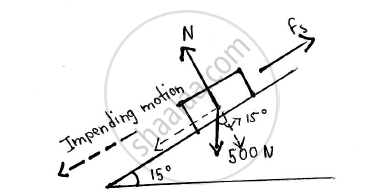Given: Weight of crate = 500 N

Initial velocity(u) = 20 m/s

μs = 0.5

μk = 0.4

θ = 15°

Final velocity (v) = 0 m/s
To find: Distance travelled by the block Time it will take before coming to rest

 Kinematics of Particle
Chapter:  Kinematics of Particle
Concept: Velocity and Acceleration in Terms of Rectangular Co-ordinate System

Derive the equation of path of a projectile and hence show that equation of path of projectile is a parabolic curve.

 Kinematics of Particle
Chapter:  Kinematics of Particle
Concept: Velocity and Acceleration in Terms of Rectangular Co-ordinate System

particle is moving in X-Y plane and it’s position is defined bybar r=(3/2 t^2)bar l+(2/3 t^3) barJ "Find radius of curvature when t=2sec."

 Kinematics of Particle
Chapter:  Kinematics of Particle
Concept: Velocity and Acceleration in Terms of Rectangular Co-ordinate System

A Force of 100 N acts at a point P(-2,3,5)m has its line of action passing through Q(10,3,4)m. Calculate moment of this force about origin (0,0,0).

 Kinematics of Particle
Chapter:  Kinematics of Particle
Concept: Velocity and Acceleration in Terms of Rectangular Co-ordinate System

State Lami’s theorem.
State the necessary condition for application of Lami’s theorem.

 Equilibrium of System of Coplanar Forces
Chapter:  Equilibrium of System of Coplanar Forces
Concept: Condition of equilibrium for parallel forces
< prev  21 to 40 of 84  next >